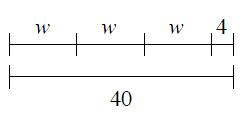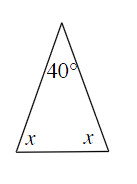### Home > MC1 > Chapter 9 > Lesson 9.2.3 > Problem9-82

9-82.

Write an equation that represents each diagram below and find the value of the variable.

1.$w + w + w + 4 = 40$
$3w + 4 = 40$
$3w = 36$
$w = 12$

1.Remember that the angles of a triangle add up to $180$ degrees.